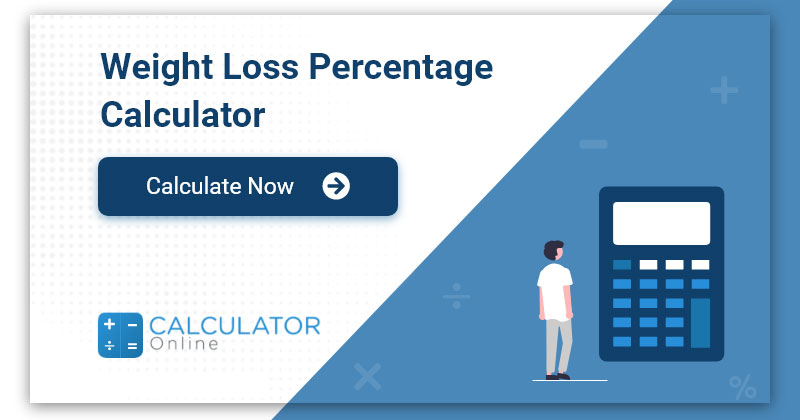# How to Calculate Percent Weight Loss

Maintaining a healthy weight is essential for a healthy lifestyle, and weight loss is often a goal for those looking to improve their overall health. However, the process of losing weight can be confusing and overwhelming, especially when it comes to calculating percent weight loss. In this article, we will explain what percent weight loss is, why it is important, and how to calculate it accurately.## What is Percent Weight Loss?

Percent weight loss is the amount of weight lost in relation to the initial weight. It is a measure of how much weight a person has lost in proportion to their starting weight. Percent weight loss is often used to track progress in weight loss programs and to assess the effectiveness of weight loss interventions.

## Why is Percent Weight Loss Important?

Calculating percent weight loss is important because it helps individuals track their progress and stay motivated. It also helps healthcare professionals monitor their patients’ progress and adjust their treatment plans accordingly. Additionally, calculating percent weight loss can help individuals set realistic weight loss goals and make informed decisions about their health.

## How to Calculate Percent Weight Loss

Calculating percent weight loss is a simple process that involves two steps:

### Step 1: Subtract the Final Weight from the Initial Weight

The first step in calculating percent weight loss is to subtract the final weight from the initial weight. For example, if your initial weight was 200 pounds and your current weight is 180 pounds, the calculation would be:

200 – 180 = 20

### Step 2: Divide the Result by the Initial Weight and Multiply by 100

The second step in calculating percent weight loss is to divide the result from step 1 by the initial weight and multiply by 100. In the example above, the calculation would be:

(20 ÷ 200) x 100 = 10%

Therefore, the percent weight loss in this example is 10%.

## Calculate Percent Weight Loss

Imperial
Metric
Basic Information
years
lbs
ft
in
Activity Level

It is important to note that percent weight loss should always be calculated using the same units of measurement. For example, if the initial weight is measured in pounds, the final weight should also be measured in pounds.

Factors that Affect Percent Weight Loss

There are several factors that can affect percent weight loss, including:

1. Initial weight: Individuals who have a higher initial weight may lose a larger amount of weight compared to those who have a lower initial weight.

2. Age: As individuals age, their metabolism slows down, which can make weight loss more difficult.

3. Gender: Men tend to lose weight faster than women due to differences in metabolism and body composition.

4. Diet and exercise: A healthy diet and regular exercise can aid in weight loss and lead to a higher percent weight loss.

5. Genetics: Genetics can play a role in weight loss and may affect an individual’s ability to lose weight.

## In conclusion

Calculating percent weight loss is a simple yet important process for anyone looking to track their weight loss progress. By following the two steps outlined above, individuals can accurately calculate their percent weight loss and use this information to set realistic weight loss goals, make informed decisions about their health, and stay motivated on their weight loss journey.